Constructing Quadrilateral - 3 Sides and 2 angles given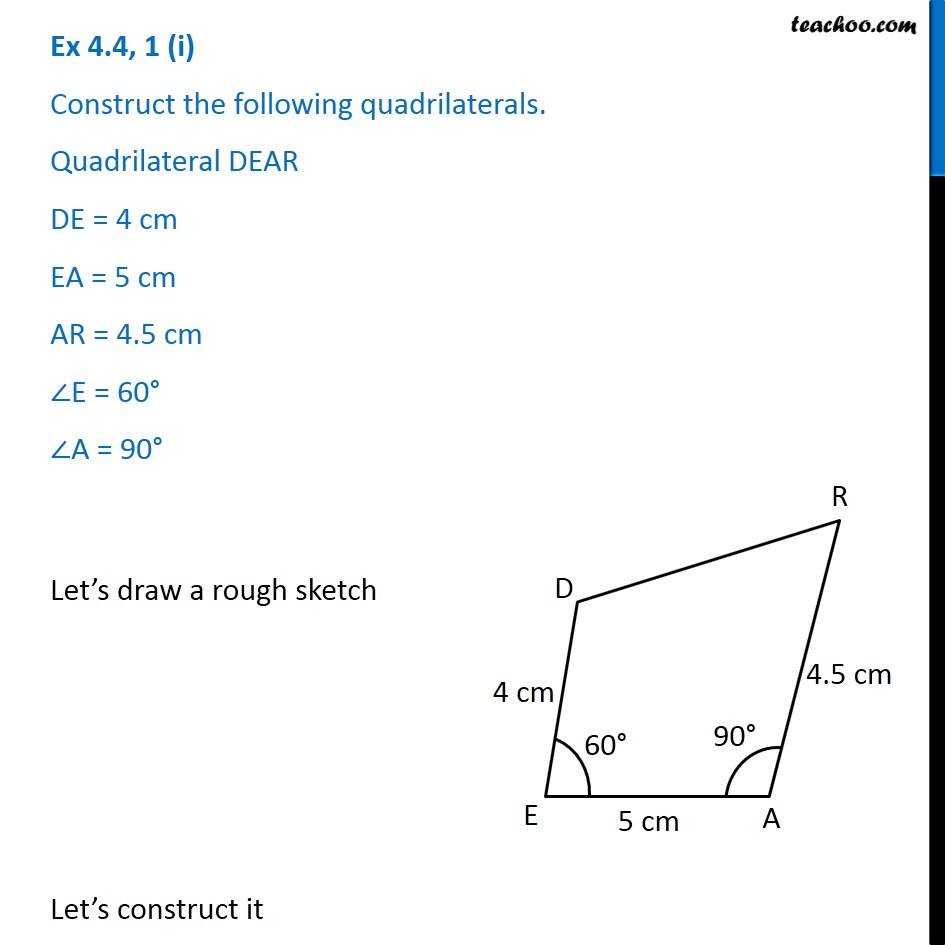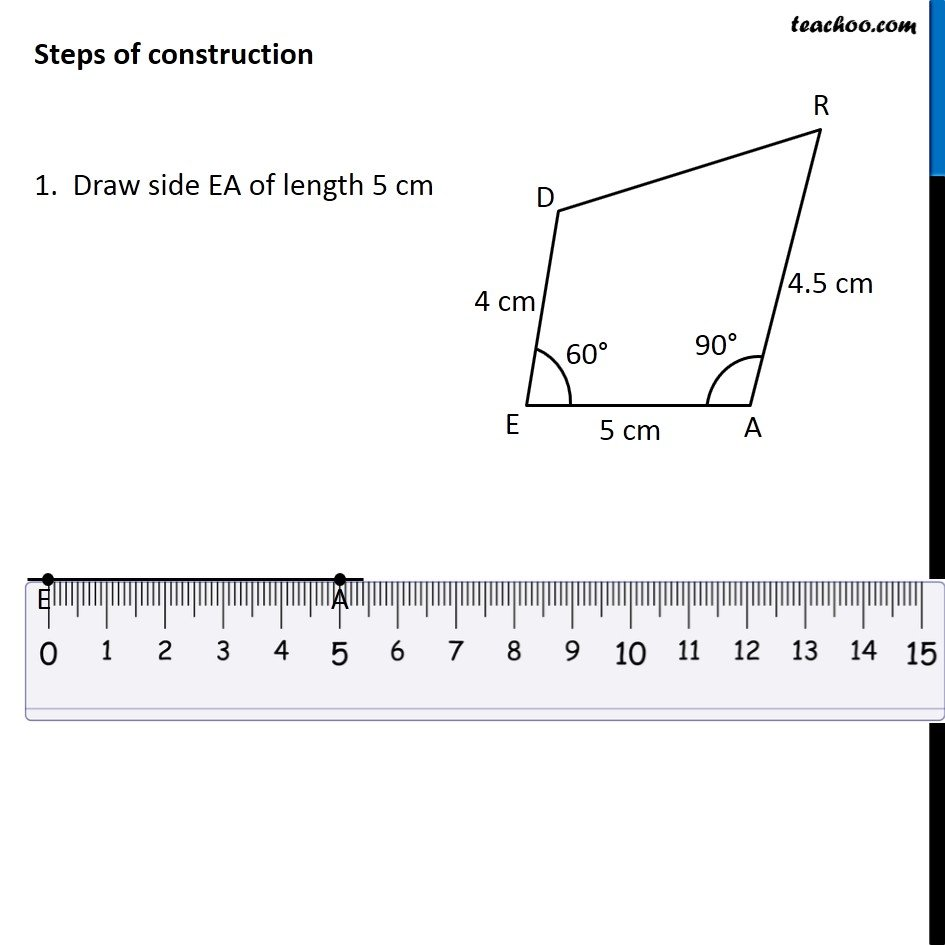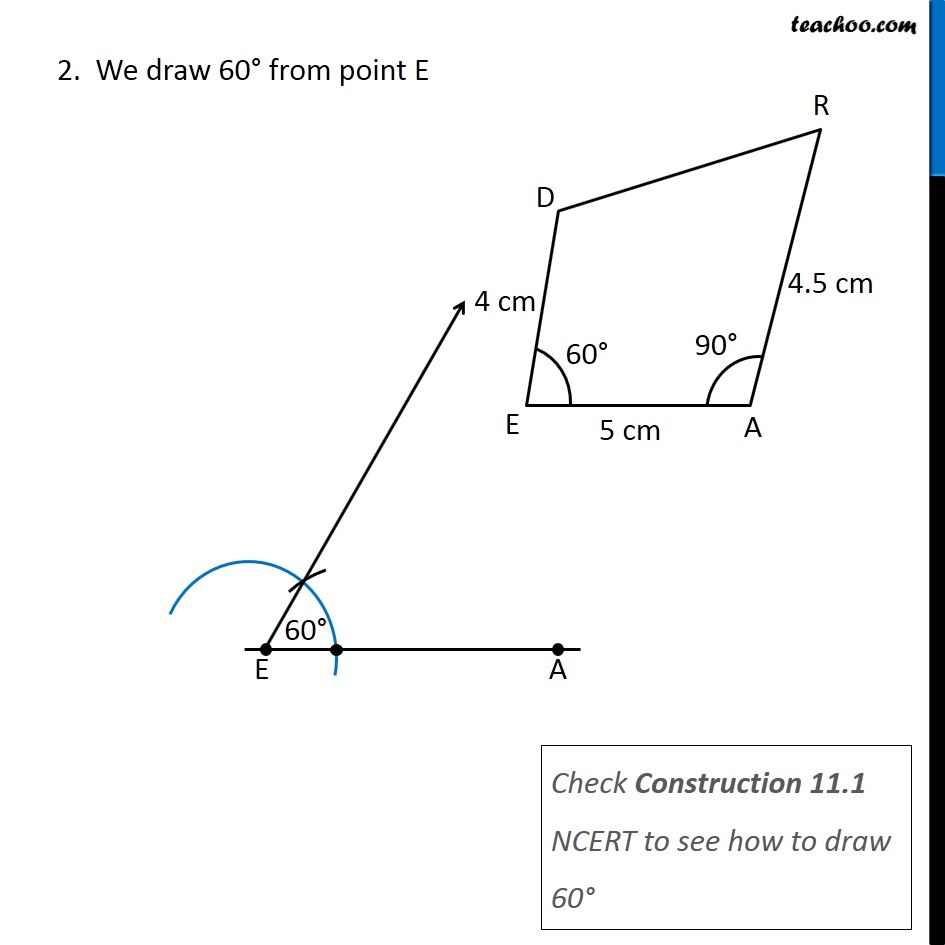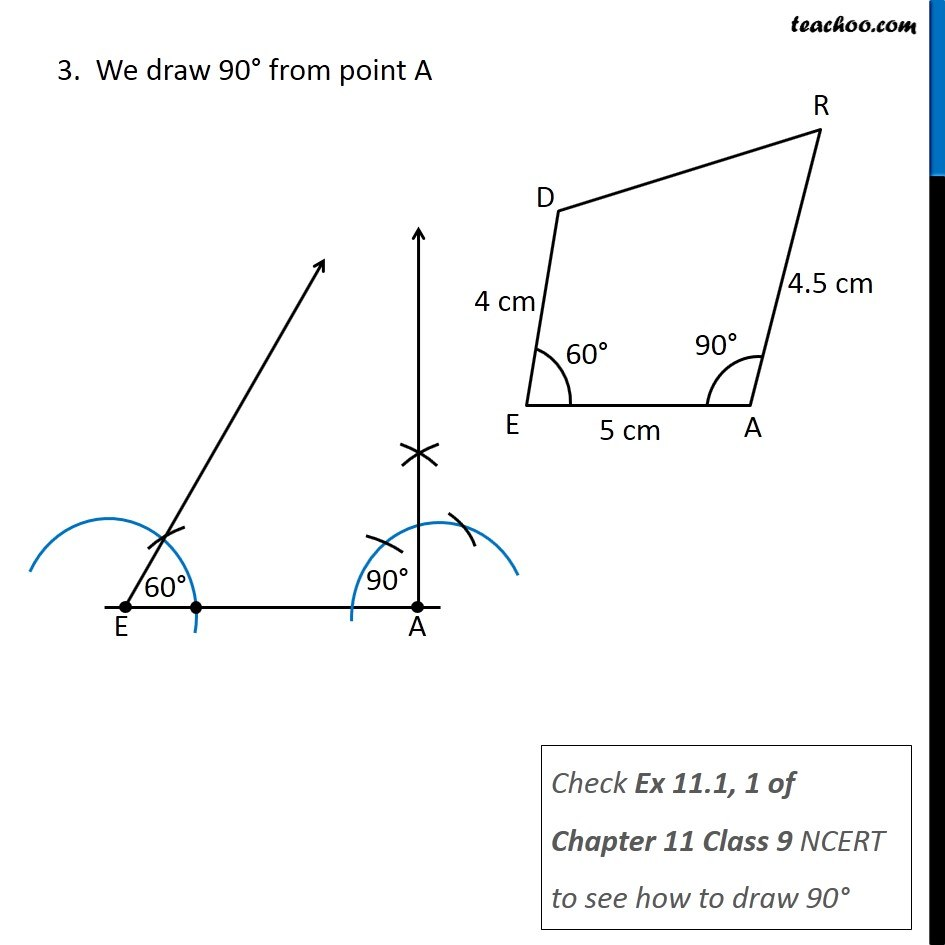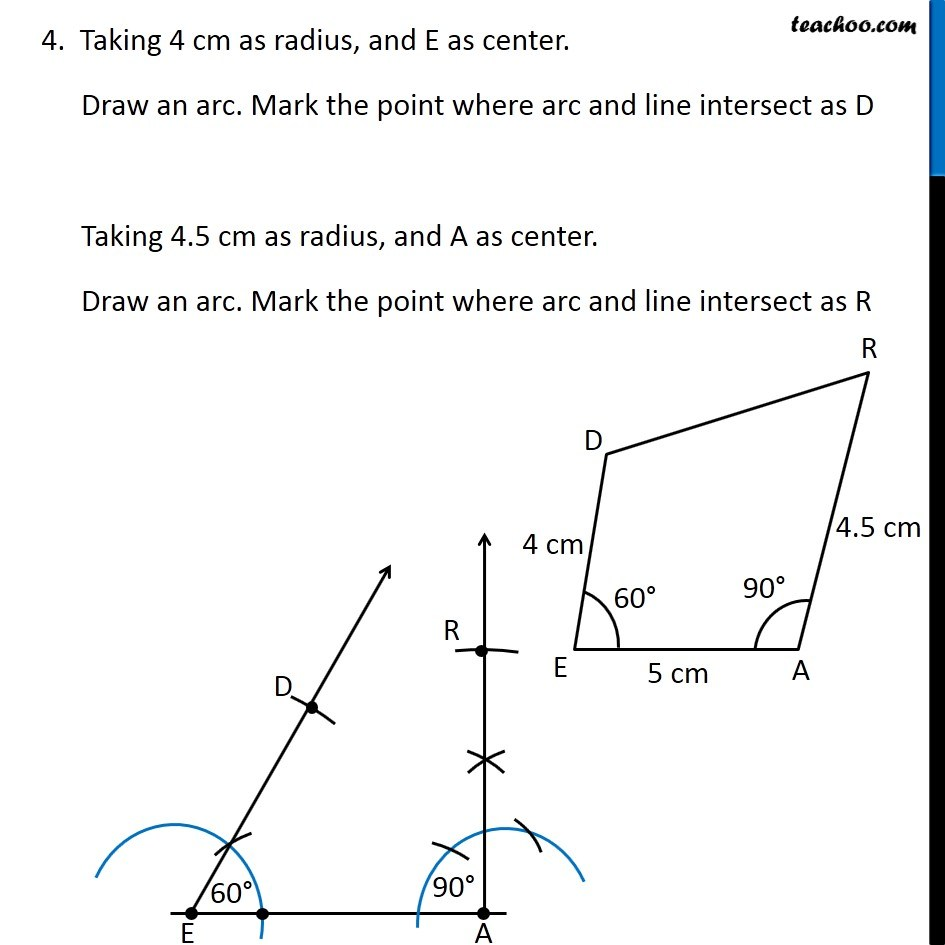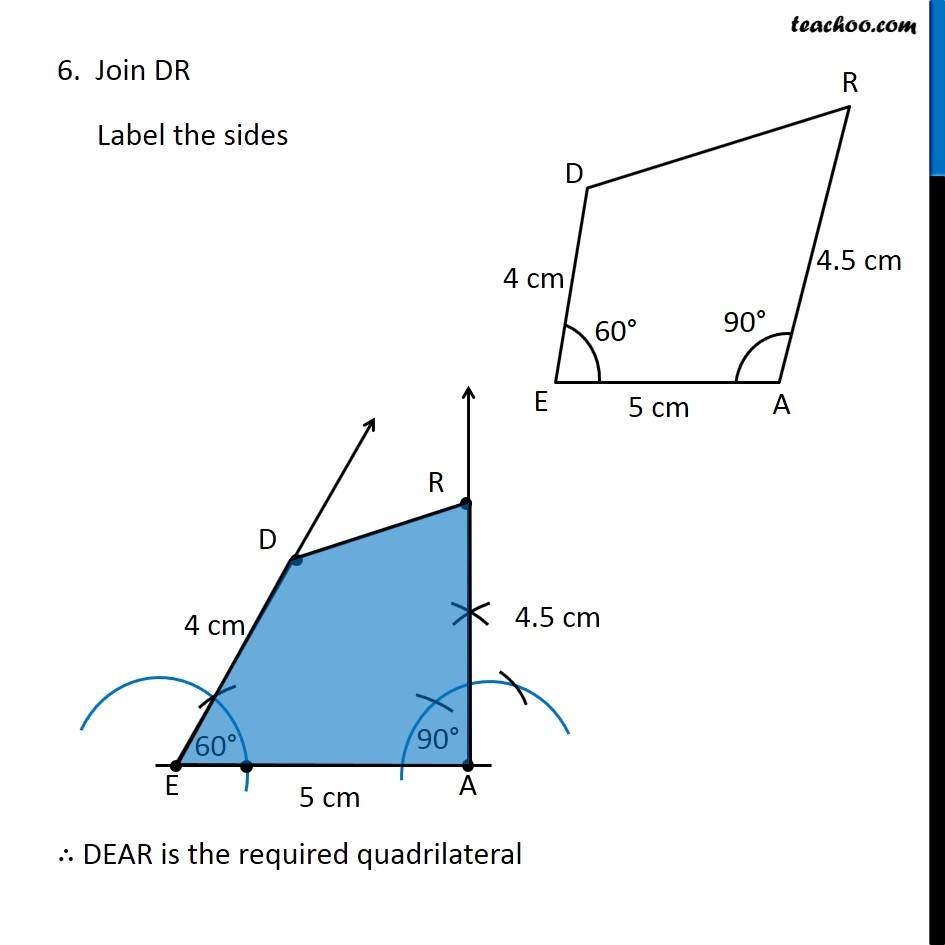Learn in your speed, with individual attention - Teachoo Maths 1-on-1 Class

### Transcript

Question 1 (i) Construct the following quadrilaterals. Quadrilateral DEAR DE = 4 cm EA = 5 cm AR = 4.5 cm ∠E = 60° ∠A = 90° Let’s draw a rough sketch Let’s construct it Steps of construction 1. Draw side EA of length 5 cm 2. We draw 60° from point E Check Construction 11.1 NCERT to see how to draw 60° 3. We draw 90° from point A Check Ex 11.1, 1 of Chapter 11 Class 9 NCERT to see how to draw 90° 4. Taking 4 cm as radius, and E as center. Draw an arc. Mark the point where arc and line intersect as D Taking 4.5 cm as radius, and A as center. Draw an arc. Mark the point where arc and line intersect as R 6. Join DR Label the sides ∴ DEAR is the required quadrilateral